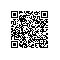# OPEN CASCADE Gauss Least Square

### OPEN CASCADE Gauss Least Square

Abstract. The least square can be used to solve a set of n linear equations of m unknowns(n >= m). The OPEN CASCADE class math_GaussLeastSquare implements the least square solution of the linear equations by using Gauss LU decomposition algorithm. The paper focus on the Least Square method to solve the linear equations.

Key Words. Least Square, LU Decomposition, Linear Equations

1.Introduction

2.Principle

math_GaussLeastSquare::math_GaussLeastSquare (const math_Matrix& A,

const Standard_Real MinPivot) :
LU(
1, A.ColNumber(),

1, A.ColNumber()),
A2(
1, A.ColNumber(),

1, A.RowNumber()),
Index(
1, A.ColNumber()) {
A2
= A.Transposed();
LU.Multiply(A2, A);

Standard_Integer Error
= LU_Decompose(LU, Index, D, MinPivot);
Done
= (!Error) ? Standard_True : Standard_False;

}

void math_GaussLeastSquare::Solve(const math_Vector& B, math_Vector& X) const{
StdFail_NotDone_Raise_if(
!Done, " ");
Standard_DimensionError_Raise_if((B.Length()
!= A2.ColNumber()) ||
(X.Length()
!= A2.RowNumber()), " ");

X.Multiply(A2, B);

LU_Solve(LU, Index, X);

return;
}

3.Code Example

/*
*    Copyright (c) 2015 Shing Liu All Rights Reserved.
*
*           File : main.cpp
*         Author : Shing Liu(eryar@163.com)
*           Date : 2015-11-25 21:00
*
*    Description : Test Gauss Least Square method to
*                  solve linear equations.
*/

#define WNT
#include
<math_GaussLeastSquare.hxx>

#pragma comment(lib,
"TKernel.lib")
#pragma comment(lib,
"TKMath.lib")

void testLeastSquare(void)
{
math_Matrix A(
1413);
math_Vector B(
14);
math_Vector X(
13);

A(
1,1= 1.0; A(1,2= 1.0; A(1,3= 0.0;  B(1= 1.0;
A(
2,1= 1.0; A(2,2= 0.0; A(2,3= 1.0;  B(2= 2.0;
A(
3,1= 1.0; A(3,2= 1.0; A(3,3= 1.0;  B(3= 0.0;
A(
4,1= 1.0; A(4,2= 2.0; A(4,3= -1.0; B(4= -1.0;

math_GaussLeastSquare aSolver(A);
aSolver.Solve(B, X);

if (aSolver.IsDone())
{
std::cout
<< aSolver;
std::cout
<< X;
}
}

int main(int argc, char* argv[])
{
testLeastSquare();

return 0;
}

4.Conclusion

5.References

1. 同济大学数学教研室. 高等数学. 高等教育出版社. 1996

2. 王仁宏. 李崇君. 朱春钢. 计算几何教程. 科学出版社. 2008

3. 罗家洪. 方卫东. 矩阵分析引论. 华南理工大学出版社. 2006

4. 易大义. 陈道琦. 数值分析引论. 浙江大学出版社. 1998

5. 赵罡. 穆国旺. 王拉柱. 非均匀有理B样条. 清华大学出版社. 2010

6. 王宜举. 修乃华. 非线性最优化理论与方法. 科学出版社. 2012使用钉钉扫一扫加入圈子
+ 订阅